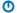# Dialog IO

Tags

Warning

Hey, [current-user:field_first_name:value]!

You haven't submitted solutions for exercises for the last lesson, Programs and variables. That'll make this lesson hard to understand. You should go back and do those exercises first.

Fill in the blank

What is it?

In programming, a _________ is a piece of memory, with a name.

Your answer:Fill in the blank

Fixed value

A ______________ is a fixed value, like 3.14, 0.41, or 512.

Your answer:### Input from cells

The tip program got its input from the worksheet, like this:Output goes to the worksheet as well. In this case, in cells B5, and B6.

So:

• Input (from cells)
• Processing
• Output (to cells)

### Input from a dialog

The program gets input from a cell, but you can get input from other places, like a dialog. A dialog is a pop-up window. For example, click this:

There was an input dialog, and then an output.

The dialog you just saw was made by the programming language JavaScript, that runs in web browsers. VBA has input dialogs, too. Suppose a worksheet was like this:When the user clicks the button:Here's the code that will do it, including variable declarations.

• Dim sMealPrice As Single
• Dim sTipAmount As Single
• Dim sTotal As Single
• ' Input meal price.
• sMealPrice = InputBox("Meal price:")
• ...

Here's the code for the earlier version, that used a worksheet cell for input:

• Dim sMealPrice As Single
• Dim sTipAmount As Single
• Dim sTotal As Single
• ' Input meal price.
• sMealPrice = Cells(1, 2)
• ...
Reflect

Compare the two code chunks. What's the same, and what's different?

(If you were logged in as a student, the lesson would pause here, and you'd be asked to type in a response. If you want to try that out, ask for an account on this site.)

Ray, what do you think?Ray

Well, they're almost the same. The variable Dim stuff is the same. So is the comment. The assignment statements even have the same left-hand-side.

• sMealPrice = Cells(1, 2)
• ...
• sMealPrice = InputBox("Meal price:")

One gets input from a cell. The other gets input from a dialog.

Right. The pattern is the same:

• Input (from a dialog)
• Processing
• Output (to dialogs)

The new program behaves differently, but has the same structure.

### Output to a dialog

We can send output to a dialog, too.The `MsgBox` function makes this type of dialog:

• MsgBox "Tip amount: " & sTipAmount

The & is called the concatenation operator. That's a geeky way of saying "put things one after the other." So put the text "Tip amount: ", and on the end of that, put the value of the variable `sTipAmount`.

Reflect

The code to output the tip amount is:

• MsgBox "Tip amount: " & sTipAmount

Write the code to output the `sTotal`.

(If you were logged in as a student, the lesson would pause here, and you'd be asked to type in a response. If you want to try that out, ask for an account on this site.)

Georgina, how would you output `sTotal`?Georgina
• MsgBox "Total: " & sTotal

Good! That's it.

### Comparing the programs

Here's the code from both versions, combined:

• Dim sMealPrice As Single
• Dim sTipAmount As Single
• Dim sTotal As Single
• ' Input.
• Plugin in either:
• Input from a cell:
• sMealPrice = Cells(1, 2)
• Or:
• Input from a dialog:
• sMealPrice = InputBox("Meal price:")
• Now the processing.
• ' Compute tip and total.
• sTipAmount = sMealPrice * 0.15
• sTotal = sMealPrice + sTipAmount
• ' Output.
• Plugin in either:
• Output to cells:
• Cells(5, 2) = sTipAmount
• Cells(6, 2) = sTotal
• Or:
• Output to dialogs:
• MsgBox "Tip amount :" & sTipAmount
• MsgBox "Total :" & sTotal

It's like the program is a template. You plug in the bits you need.

### Fewer dialogsAdela

In the new version of the program, the one with the dialogs. We have two output dialogs.Could we combine the output? Like this:Sure. That's a good idea, since it puts all of the output together. Replace these lines...

• MsgBox "Tip amount: " & sTipAmount
• MsgBox "Total: " & sTotal

... with this...

• MsgBox "Tip amount: " & sTipAmount & vbCrLf & "Total: " & sTotal

`vbCrLf` tells VBA to start a new line. It's like hitting Enter in the output.

### Long lines of code

Here's that line again:

• MsgBox "Tip amount: " & sTipAmount & vbCrLf & "Total: " & sTotal

If a line of code is getting too long, you can break it up:

• MsgBox "Tip amount: " & sTipAmount & vbCrLf _
•   & "Total: " & sTotal

The underscore is VBA's line continuation character. It lets you break up a long line of code, to make it easier to read. You don't have to use it, but it helps sometimes.

### Up next

What happens when users do things we don't expect?